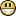Improved support for KaTeX (Possible bug?)

Hello,

We have a DocRaptor customer who's provided a sample document using KaTeX that renders inline fractions with additional offsets from the top vs alignment being perfect in web browsers.

They've been kind enough to provide a minimal example below. (Since we use PhantomJS to process most JavaScript documents in DocRaptor, I'm attaching the JS-pre-processed HTML and PDF as well.)

<!DOCTYPE html>
<html>
<!-- This tells Prince what character set we are using. Don't remove it! -->
<meta http-equiv="Content-Type" content="text/html; charset=utf-8" />
<meta http-equiv="X-UA-Compatible" content="IE=edge,chrome=1">
<title>KATEX TEST</title>
<meta name="viewport" content="width=device-width">
<script src="https://cdn.jsdelivr.net/npm/katex@0.13.0/dist/katex.min.js"></script>
<script>
var finishedProcessing = false;

function runKatexPostProcessing() {
var eles = document.querySelectorAll(".katex-eq:not(.katex-rendered)");

for (idx = 0; idx < eles.length; idx++) {
var ele = eles[idx];

try {
katex.render(
ele.textContent,
ele,
{
displayMode: ele.getAttribute("data-katex-display") === 'true',
throwOnError: false
}
);
} catch (n) {
ele.style.color = "red";
ele.textContent = n.message;
}
}

setTimeout(function() {
finishedProcessing = true;
}, 3500);
}

function docraptorJavaScriptFinished() {
return finishedProcessing;
}

</script>
<style type="text/css">
/* Katex Overrides */
.katex-eq {
display: block;
margin-bottom: 1.5rem;
}
</style>
<body>
<span class="katex-eq" data-katex-display="false">Writes\ per\ second= \left[ ~\frac{A}{2} \times \sin sin~ \left( ~\frac{2 \pi x}{f}~- \frac{ \pi }{2}~ \right) +b+ \frac{A}{2}~ \right] \times R_{ \sigma =0.5}^{ \mu =1} \times 0.3</span>

<span class="katex-eq" data-katex-display="false">Reads\ per\ second=  \left[ ~\frac{A}{2}  \times \sin sin~ \left( ~\frac{2 \pi x}{f}~-  \frac{ \pi }{2}~ \right)  +b+ \frac{A}{2}~ \right]   \times  R_{ \sigma =0.5}^{ \mu =1}</span>
</body>
</html>

We've added a timeout just in case KaTeX needed more time for layout passes (MathJax does sometimes), but that doesn't seem to be the case here.

I was wondering if there was a solution to this anyone can think of (other than the manual overrides we're doing now) or if there's some CSS or other rendering changes that would be needed on the PrinceXML side to render this the same way as is done in web browsers.

Best,
Alex
DocRaptor Support
mikeday
Thanks we will investigate this issue.
mikeday
Today we have released Prince 14.2 with a fix for this table vertical align issue, thanks again for letting us know!